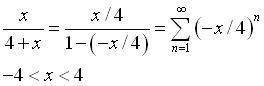# Power Series Representation

kskiraly

## Homework Statement

Find a power series representation for the function

f(x) = x / (4+x)

and determine the interval of convergence.

I have no idea how to begin this problem.

My only guess would be trying to divide something out in order to simplify to something that I'm able to create something of the form (x+c)^n

I can work out to the interval of convergence, I am just unsure of how to create the representation.

## Answers and Replies

Homework Helper
Gold Member
Try this one:It is a geometric series, so I am not so sure that it is the correct answer to your question.

#### Attachments

christianjb
Taylor series expansion about x=0. Substitute expression for the nth derivative of f(x) evaluated at x=0.

The answer comes to

f(x)=-sum (-x/4)^n

where the sum is from 0 to infinity

Homework Helper
Malawi glenn was steering you right! The geometric sequence $\sum_{n=0}^\infty ar^n$ converges to $\frac{a}{1- r}$. Now what must a and r be in order to converge to
[tex]\frac{x}{4+ x}= \frac{\frac{x}{4}}{1-(-\frac{x}{4})}}[/itex]? For what values of r does a geometric series converge?

Yes, you could do this by differentiating and forming the Taylor's series but that is tedious with lots of room for error. And, in fact, the answer given by christianjb is incorrect: For x= 0, "f(x)=-sum (-x/4)^n,where the sum is from 0 to infinity" is 1 while x/(1+ x) is 0.

Homework Helper
Rewrite as $x\cdot \frac{1}{x+4}$ and use long division.

christianjb
Malawi glenn was steering you right! The geometric sequence $\sum_{n=0}^\infty ar^n$ converges to $\frac{a}{1- r}$. Now what must a and r be in order to converge to
[tex]\frac{x}{4+ x}= \frac{\frac{x}{4}}{1-(-\frac{x}{4})}}[/itex]? For what values of r does a geometric series converge?

Yes, you could do this by differentiating and forming the Taylor's series but that is tedious with lots of room for error. And, in fact, the answer given by christianjb is incorrect: For x= 0, "f(x)=-sum (-x/4)^n,where the sum is from 0 to infinity" is 1 while x/(1+ x) is 0.

I should have said n=1,infiniy. Then the answer is correct.

Homework Helper
I should have said n=1,infiniy. Then the answer is correct.

Yes. Minor detail but that's the point- calculating the Taylor's series leaves a lot of room for error!

christianjb
Yes. Minor detail but that's the point- calculating the Taylor's series leaves a lot of room for error!

I agree that the geometric series method is more elegant in this case.